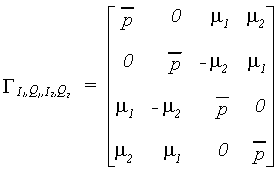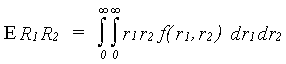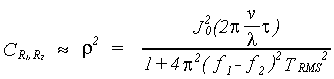### JPL's Wireless Communication Reference Website

#### Chapter: Wireless Propagation Channels Section: Multipath Fading, Rayleigh fading, Coherence Bandwidth# Coherence Bandwidth

Consider two (random) sinusoidal signals, with sample 1 taken at frequency f1 at time t1 and sample 2 at frequency f2 at time t2. The in-phase and quadrature components I(t) and Q(t of one sample are i.i.d. Gaussian. Also, I1, Q1, I2, Q2 is a jointly Gaussian random vector. The covariance matrix of (I1, Q1, I2, Q2) isfor the most general case, i.e., with non-zero time difference t = t2 - t1, we haveandwhere J0 is the Bessel function of first kind of order 0, and TRMS is the rms delay spread. Note that the Bessel function J0(0) = 1 for zero time differences. We now have to convert I and Q to amplitudes R.

### Envelope Correlation (Amplitudes)

The amplitude is found from R12 = I12 + Q12. We convert from (I1, Q1, I2, Q2) to (R1, f1, R2, f2). The associated Jacobian is ˝J˝ = R1 R2 So the PDF is

f(r1,f1,r2,f2) = r1r2 f(i1=r1cos f1, q1=r1sinf1, i2=r2cosf2, q2=r2sinf2).

Integrating over f1 and f2 giveswhere the Bessel function I0 occurs due to ň exp{cosf} df

The normalized correlation coefficient r is## Envelope Correlation

We now take the expected value E R1R2Inserting the PDF (with Bessel function) gives the Hypergeometric integralThis integral can be expanded asMostly, only the first two terms are considered

## Normalized Envelope Covariance

The normalized covariance takes on values in the interval 0Ł C Ł1

It is defined asHere we have that

 Local-mean value: ER1 = Ö(p/ 2) Variance: VARR1 = SIG2 R1 = (2 - p / 2) Correlation: ER1R2 » p /2 [1 + r2 / 4]
Thus, after some algebra,